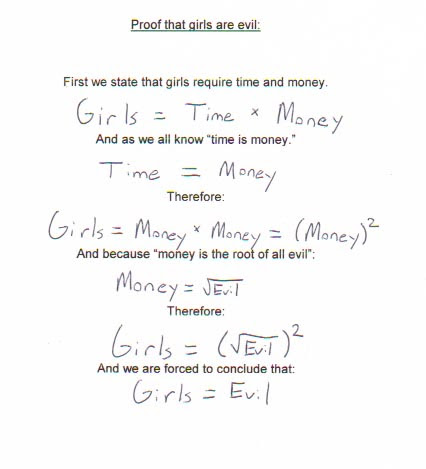## Wednesday, January 27, 2010

### Entropic Everything

The latest paper by Eric Verlinde on gravity as an entropic force makes me wonder whether I am getting old: Let me admit it: I just don't get it. Is this because I am conservative or lack imagination or too narrow minded? If it were not for the author, I would have rated it as pure crackpottery. But maybe I am missing something. Today, there were three follow-up papers dealing with cosmological consequences (the idea being roughly that Verlinde uses the equipartition of energy between degrees of freedom each getting a share of 1/2 kT which is not true quantum mechanically at low temperatures as there the system is in the ground state with the ground state energy. As in this business temperature equals acceleration a la Unruh this means the argument is modified for small accelerations which is a modification of MOND type).

Maybe later I try once more to get into the details and might have some more sensible comments then but right now the way different equations from all kinds of different settings (Unruh temperature was already mentioned, E=mc^2, one bit per Planck area, etc) are assembled reminds me of this:## Tuesday, January 19, 2010

### Instability of the QED vacuum at large fine structure constant

Today, in the "Mathematical Quantum Mechnics" lecture, I learned that the QED vacuum (or at least the quantum mechanical sector of it) is unstable when the fine structure constant gets too big.

To explain this, let's go back to a much simpler problem: Why is the hydrogen-like atom stable? Well, a simple answer is that you just solve it and find the spectrum to be bounded above. But this answer does not extend to other problems that cannot be diagonalised analytically.

First of all, what is the problem we are considering? It's the potential energy of the electron which in natural (for atomic physics) units is. And this goes to negative infinity whengoes to 0. But quantum mechanics saves you. Roughly speaking (this argument can be made mathematically sound in terms of Hardy's inequality), if you essentially localise the electron in a ball of radiusand thus have the potential energy, Heisenberg's uncertainty implies the momentun is at least of the orderand thus the kinetic energy is at least of the order. Thus, whenbecomes small and you seem to approach the throat of the potential the positive kinetic energy wins and thus the Hamiltonian of the hydrogen atom is bounded from below. This is the non-relativistic story.

Close to the nucleus however, the momentum can be so big that you have to think relativistically. But then trouble starts as at large momenta the energy grows only linearly with momentum and thus the kinetic energy only scales likewhich is the same as the potential energy. Thus a more careful calculation is needed. The result of it is that it depends onwhich term eventually wins. Above a critical value (which happens to be of order one) the atom is unstable and one can gain an infinite amount of energy by lowering the electron into the nucleus and quantum mechanics is not going to help.

Luckily, nuclei with large enoughdo not exist in nature. Well, with the exception of neutron stars which are effectively large nuclei. And there it happens. All the electrons are sucked into the nuceus and fuse with the protons to neutrons. In fact, the finite size of the nucleon is what regulates this process as thenature of the Coulomb potential is smeared out in the nucleus. But such a highly charged atom would be only of the size of the nucleus (about some femto meters) rather than the size of typical atoms.

But now comes QED with the possibility of forming electron-positron pairs out of the vacuum. The danger I am talking about is the fact that they can form a relativistic, hydrogen like bound state. And both are (as far as we know) point like and thus there is no smearing out of the charge. It is only thatin this case which luckily is less than one. If it would be bigger you could create this infinte amount of energy from the vacuum by pair creation and bringing them on-shell in their relative Coulomb throat. What a scary thought. Especially, sinceis probably only the vev of some scalar field which can take other values in other parts of the multiverse which would then disappear with a loud bang.

Some things come to my mind which in principle could help but which turn out to make things worse:is not a constant but it's running and QED has asymptotic slavery which means at short distances (which we are talking about) it gets bigger and makes things worse. Further, we are treating the electromagnetic field classically which of course is not correct. But my mathematical friends tell me that quantizing it also worsens things.

We know, QED has other problems like the Landau pole (a finite scale wheregoes to infinity due to quantum effects). But it seems to me that this is a different problem since it already appears at.